﻿ Energy Stored in a Magnetic FieldBack

## Energy Stored in a Magnetic Field# Energy Stored in a Magnetic Field

Magnetic field can be of permanent magnet or electro-magnet. Both magnetic fields store some energy. Permanent magnet always creates the magnetic flux and it does not vary upon the other external factors. But electromagnet creates its variable magnetic fields based on how much current it carries. The dimension of this electro-magnet is responsible to create the strength the magnetic field and hence the energy stored in this electromagnet.

First we consider the magnetic field is due to electromagnet i.e. a coil of several no. turns. This coil or inductor is carrying current I when it is connected across a battery or voltage source through a switch.Suppose battery voltage is V volts, value of inductor is L Henry, and current I will flow at steady state.

When the switch is ON, a current will flow from zero to its steady value. But due to self induction a induced voltage appears which isthis E always in the opposite direction of the rate of change of current.Now here the energy or work done due to this current passing through this inductor is U.

As the current starts from its zero value and flowing against the induced emf E, the energy will grow up gradually from zero value to U.dU = W.dt, where W is the small power and W = - E.I

So, the energy stored in the inductor is given by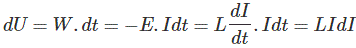Now integrate the energy from 0 to its final value.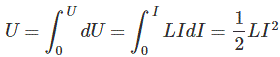Again,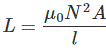as per dimension of the coil, where N is the number of turns of the coil, A is the effective cross-sectional area of the coil and l is the effective length of the coil.

Again,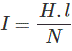Where, H is the magnetizing force, N is the number of turns of the coil and l is the effective length of the coil.Now putting expression of L and I in equation of U, we get new expression i.e.So the stored energy in a electromagnetic field i.e. a conductor can be calculated from its dimension and flux density.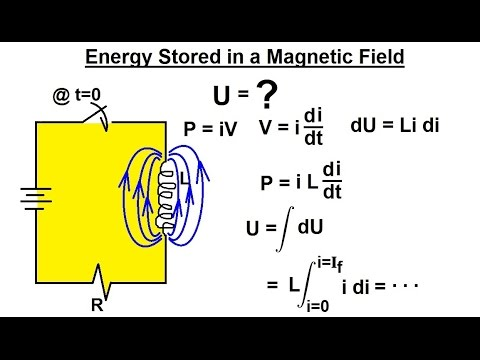Now let us start discussion about energy stored in the magnetic field due to permanent magnet.

Total flux flowing through the magnet cross-sectional area A is φ.

Then we can write that φ = B.A, where B is the flux density.

Now this flux φ is of two types, (a) φr this is remanent flux of the magnet and (b) φd this is demagnetizing flux.

So,as per conservation of the magnetic flux Law.Again, Bd = μ. H, here H is the magnetic flux intensity.

Now MMF or Magneto Motive Force can be calculated from H and dimension of the magnet.where l is the effective distance between two poles.Now to calculate energy we have to first go for the reluctance of the magnetic flux path.

Magnet’s internal reluctance path that is for demagnetizing is denoted as Rm,

And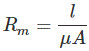Now Wm, is the energy stored in the magnet's internal reluctance.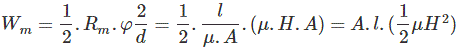Now energy densityLook at the model below. Dotted lined box is the magnet and one reluctance path Rl for the mechanical load is connected across the magnet.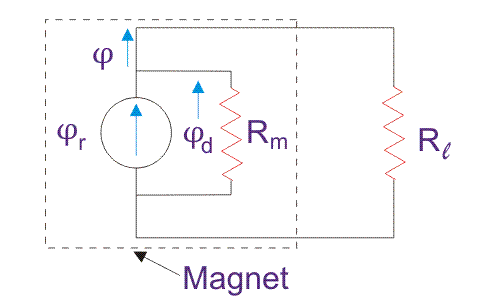Now apply node equation and loop equation, we getNow, If we do any mechanical work inside a magnetic field, then the energy required W.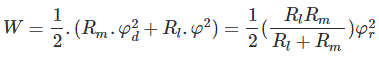Again, if we place a electromagnetic coil in the vicinity of a permanent magnet, then this coil will experience a force. To move this coil some work is done. This energy density is the co-energy with respect to the permanent magnet and the coil magnet. Magnetizing flux intensity for the permanent magnet is H and for the coil is HC .

This co-energy is denoted as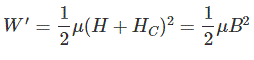Where, B is the flux density at the coil position near the permanent magnet.